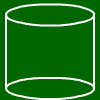# Calculator for cylinders

Cylinder formulas:
Base area = pi * radius²
Volume = base area *height
Lateral area = perimeter * height
Surface = 2 * base area + lateral area

## What is a cylinder?

A cylinder is gotten by connecting two circles lying one above the other.

## What are the cylinder formulas?

The base area is pi*r² cause it is a circle, and for the same reason the base area perimeter is 2*pi*r. The lateral area equals U*h where U is the base area perimeter. The surface is 2*G+M where G is the base area and M the lateral area. The volume is G*h.Radius base area, base area diameter
base area perimeter
base area
height
lateral area surface
volume